• 利用Python集成了20多常用的图像处理方法包括二值化、边缘检测、开闭运算、高斯模糊、直方图等操作。仅需要读取图片便能运行，可在Python3环境下直接运行，无需调整。
• 总结了matlab中最常用的数字图像处理函数的使用方法包括灰度化，二值化，中值滤波，图像变换，图像增强等的实例。matlab
• 介绍了各种图像处理的常用方法包括图像平移、图像颠倒、图像镜像变换、图像旋转
• 使用opencv 进行图像特效的处理包括 灰度图像的处理（基础），图像颜色反转，图像马赛克效果，图像毛玻璃效果，图像边缘检测，图像融合，浮雕效果，颜色映射效果，油画特效，使用opencv 3 python版本 3.6 ...

使用opencv 进行图像特效的处理，包括 灰度图像的处理（基础），图像颜色反转，图像马赛克效果，图像毛玻璃效果，图像边缘检测，图像融合，浮雕效果，颜色映射效果，油画特效，使用opencv 3 python版本 3.6

01 图像灰度处理
# 使用 imread 方法直接读取灰白的图形

import cv2
img=cv2.imread('img.jpg',0)
cv2.imshow('gray image',img)
cv2.waitKey(0)

-1

# 使用cvtColor API进行转换
import cv2
img=cv2.imread('img.jpg',1)
# 颜色空间转换：第一个参数是图像对象，第二个参数是颜色转换的方式：
# 例子中颜色转换的方式是 ： 从BGR转换到GRAY
new_img=cv2.cvtColor(img,cv2.COLOR_BGR2GRAY)

cv2.imshow('gray image',new_img)
cv2.waitKey(0)

-1

# 使用灰度图像原理来实现图像的灰度处理：
#  R = G = B = Gray  Gray=(R+G+B)/3
import cv2
import numpy as np
img=cv2.imread('img.jpg',1)
imageInfo=img.shape
height=imageInfo
width=imageInfo
new_img=np.zeros(imageInfo,np.uint8)
for i in range(0,height):
for j in range(0,width):
(b,g,r)=img[i,j]
gray=(int(b)+int(g)+int(r))/3
new_img[i,j]=np.uint8(gray)

cv2.imshow('gray image',new_img)
cv2.waitKey(0)

-1

# 使用灰度处理算法原理进行图像灰度的处理：
#  gray =r*0.299 + g*0.587 + b* 0.114
import cv2
import numpy as np
img=cv2.imread('img.jpg',1)
imageInfo=img.shape
height=imageInfo
width=imageInfo
new_img=np.zeros(imageInfo,np.uint8)
for i in range(0,height):
for j in range(0,width):
(b,g,r)=img[i,j]
r=int(r)
g=int(g)
b=int(b)
gray=r*0.299+g*0.587+b*0.114
new_img[i,j]=np.uint8(gray)

cv2.imshow('gray image',new_img)
cv2.waitKey(0)

-1

# 算法优化
# 尽量避免使用浮点运算，用顶点运算进行替换
# 移位运算要比 加减乘除都要快
# gray=0.299*r+0.587*g+0.114*b 可以转化为： （r+g/2+b)/4 舍弃了一些精度
# 进一步优化： gray=(r+(g<<1)+b)>>2
import numpy as np
import cv2
img=cv2.imread("img.jpg",1)
imageInfo=img.shape
height=imageInfo
width=imageInfo
mode=imageInfo
new_img=np.zeros(imageInfo,np.uint8)
for i in range(0,height):
for j in range(0,width):
b,g,r=img[i,j]
r=int(r)
g=int(g)
b=int(b)
gray=(r+(g<<1)+b)>>2
new_img[i,j]=gray
cv2.imshow("image",new_img)
cv2.waitKey(0)

-1

02 图像颜色反转
# opencv 图像颜色反转
# 灰度图像颜色的反转
import cv2
import numpy as np

img=cv2.imread("img.jpg",1)
imageInfo=img.shape

width=imageInfo
height=imageInfo
mode=imageInfo

new_img=np.zeros((height,width,1),np.uint8)
gray=cv2.cvtColor(img,cv2.COLOR_BGR2GRAY)

for i in range(0,height):
for j in range(0,width):
temp=gray[i,j]
new_img[i,j]=255-temp
cv2.imshow("before",img)
cv2.imshow("gray",gray)
cv2.imshow("after",new_img)
cv2.waitKey(0)

# opencv 图像反转
# 彩色图像的颜色反转
import cv2
import numpy as np

img=cv2.imread("img.jpg",1)
imageInfo=img.shape

width=imageInfo
height=imageInfo
mode=imageInfo

new_img=np.zeros((height,width,3),np.uint8)
for i in range(0,height):
for j in range(0,width):
(b,g,r)=img[i,j]
new_img[i,j]=(255-b,255-g,255-r)
cv2.imshow("before",img)
cv2.imshow("after",new_img)
cv2.waitKey(0)

-1

图片马赛克效果
import cv2
img=cv2.imread("img.jpg",1)
imageInfo=img.shape
height=imageInfo
width=imageInfo
mode=imageInfo

print(imageInfo)

#设定马赛克范围
#  x (150--->250)
#  y (280--->380)

for i in range(150,250):
for j in range(280,380):
# 马赛克的原理就是在一个点 取一个颜色值，然后在一定的范围之内都是这个颜色值。
# 这里选定每 （10 x 10） 的方块为一个马赛克块。
if i%10==0 and j%10==0:
(b,g,r)=img[i,j]
for row in range(10):
for col in range(10):
img[row+i,j+col]=(b,g,r)
cv2.imshow("after",img)
cv2.waitKey(0)

(375, 500, 3)

-1

图片毛玻璃效果
import cv2
import random
import numpy as np
img=cv2.imread("img.jpg",1)
imageInfo=img.shape
height=imageInfo
width=imageInfo
mode=imageInfo

new_img=np.zeros(imageInfo,np.uint8)
mm=8
for i in range(0,height-mm):
for j in range(0,width-mm):
index=int(random.random()*mm)
(b,g,r)=img[i+index,j+index]
new_img[i,j]=(b,g,r)
cv2.imshow("new image",new_img)
cv2.waitKey(0)

-1

图片融合
# 图片融合实际上就是两张图片各自保留多少透明度，然后叠加在一起形成的图片
import cv2
import numpy as np
img=cv2.imread("image.png",1)
img1=cv2.imread("img.jpg",1)

info1=img.shape
info2=img1.shape
print(info1)
print(info2)

# 定义新图像的高和宽

height=300
width=400
img_temp=img[0:height,0:width]
img_temp1=img1[0:height,0:width]
new_img=np.zeros((height-1,width-1,3),np.uint8)
new_img=cv2.addWeighted(img_temp,0.5,img_temp1,0.5,0)
cv2.imshow("image 1",img)
cv2.imshow("image 2",img1)
cv2.imshow("new image",new_img)
cv2.waitKey(0)

(678, 1464, 3)
(375, 500, 3)

-1

边缘检测
# 边缘检测实际上是图像的卷积运算
# 通过边缘检测，可以把图片明显的边缘显示出来。平滑的部分是黑色
import cv2
import random
import numpy as np
img=cv2.imread("img.jpg",1)
gray=cv2.cvtColor(img,cv2.COLOR_BGR2GRAY)
# 滤波功能：
imgG=cv2.GaussianBlur(gray,(3,3),0)
# 调用Canny 方法实现边缘检测
new_img=cv2.Canny(img,50,50)
cv2.imshow("after",new_img)
cv2.waitKey(0)

-1

浮雕效果
import cv2
import random
import numpy as np
img=cv2.imread("img.jpg",1)
imageInfo=img.shape
height=imageInfo
width=imageInfo
mode=imageInfo

gray=cv2.cvtColor(img,cv2.COLOR_BGR2GRAY)

new_img=np.zeros((height,width,1),np.uint8)
for i in range(height):
for j in range(width-1):
grayP0=int(gray[i,j])
grayP1=int(gray[i,j+1])
newP=grayP0-grayP1+150
if newP>255:
newP=25
if newP<0:
newP=0
new_img[i,j]=newP
cv2.imshow("dst",new_img)
cv2.waitKey(0)

-1

颜色映射
# 颜色增强效果，这里实现的是加强蓝色
# 使用公式  b*1.5>255? 255:b*1.5
# g*1.3>255? 255:g*1.3
import cv2
import numpy as np
img=cv2.imread("img.jpg",1)
new_img=np.zeros(img.shape,np.uint8)
imageInfo =img.shape
height=imageInfo
width=imageInfo

for i in range(0,height):
for j in range(0,width):
(b,g,r)=img[i,j]
b=b*1.5
g=g*1.3
if b>255:
b=255
if g>255:
g=255
new_img[i,j]=(b,g,r)
cv2.imshow("old image",img)
cv2.imshow("new image",new_img)
cv2.waitKey(0)

-1

油画特效
# 油画特效的原理是用一个小方块中多数的灰度值表示当前块内所有的灰度值
# 用肉眼看的话直观的感觉是没有原图纹理清晰，也就是油画的特点
# 步骤： 1 图片灰度处理 2 图片灰度等级划分 3 遍历每一个块，统计出占比比较大的灰度等级 K 4 将 将原图中满足 K灰度等级的一个像素取出作为
# 目标图像的颜色值。
import cv2
import numpy as np
# 运算量比较大，要等一会才会出结果。
img=cv2.imread("img.jpg",1)
imageInfo=img.shape
height=imageInfo
width=imageInfo
mode=imageInfo
#灰度转换
gray=cv2.cvtColor(img,cv2.COLOR_BGR2GRAY)

new_img=np.zeros((height,width,3),np.uint8)

# 这里定义方格大小为 8 x 8
# 定义灰度等级为 8 个级别 即：0-255 共 256 个，分成八个等级，每个等级32个灰度，例如 31 处于第一级，50处于第二级
for i in range(4,height-4):
for j in range(4,width-4):
array1=np.zeros(8,np.uint8)
# 遍历小方块，统计方块中的灰度值
for m in range(-4,4):
for n in range(-4,4):
index=int(gray[i+m,j+n]/32)
array1[index]=array1[index]+1
MaxLeval=array1
l=0
for k in range(0,8):
if MaxLeval=(l*32) and gray[i+m,j+n]<=((l+1)*32):
(b,g,r)=img[i+m,j+n]
new_img[i,j]=(b,g,r)

cv2.imshow("new image",new_img)
cv2.waitKey(0)

-1



展开全文opencv python
•  （一）特点：各种基于形状特征检索方法都可以比较有效地利用图像中感兴趣目标来进行检索，但它们也有一些共同问题，包括：①目前基于形状检索方法还缺乏比较完善数学模型；②如果目标有变形时检索结果...

形状特征
（一）特点：各种基于形状特征的检索方法都可以比较有效地利用图像中感兴趣的目标来进行检索，但它们也有一些共同的问题，包括：①目前基于形状的检索方法还缺乏比较完善的数学模型；②如果目标有变形时检索结果往往不太可靠；③许多形状特征仅描述了目标局部的性质，要全面描述目标常对计算时间和存储量有较高的要求；④许多形状特征所反映的目标形状信息与人的直观感觉不完全一致，或者说，特征空间的相似性与人视觉系统感受到的相似性有差别。另外，从
2-D 图像中表现的 3-D 物体实际上只是物体在空间某一平面的投影，从 2-D 图像中反映出来的形状常不是 3-D
物体真实的形状，由于视点的变化，可能会产生各种失真。
（二）常用的特征提取与匹配方法
Ⅰ几种典型的形状特征描述方法
通常情况下，形状特征有两类表示方法，一类是轮廓特征，另一类是区域特征。图像的轮廓特征主要针对物体的外边界，而图像的区域特征则关系到整个形状区域。
几种典型的形状特征描述方法：
（1）边界特征法该方法通过对边界特征的描述来获取图像的形状参数。其中Hough
变换检测平行直线方法和边界方向直方图方法是经典方法。Hough
变换是利用图像全局特性而将边缘像素连接起来组成区域封闭边界的一种方法，其基本思想是点—线的对偶性；边界方向直方图法首先微分图像求得图像边缘，然后，做出关于边缘大小和方向的直方图，通常的方法是构造图像灰度梯度方向矩阵。
（2）傅里叶形状描述符法
傅里叶形状描述符(Fourier
shape
descriptors)基本思想是用物体边界的傅里叶变换作为形状描述，利用区域边界的封闭性和周期性，将二维问题转化为一维问题。
由边界点导出三种形状表达，分别是曲率函数、质心距离、复坐标函数。
（3）几何参数法
形状的表达和匹配采用更为简单的区域特征描述方法，例如采用有关形状定量测度（如矩、面积、周长等）的形状参数法（shape
factor）。在 QBIC
系统中，便是利用圆度、偏心率、主轴方向和代数不变矩等几何参数，进行基于形状特征的图像检索。
需要说明的是，形状参数的提取，必须以图像处理及图像分割为前提，参数的准确性必然受到分割效果的影响，对分割效果很差的图像，形状参数甚至无法提取。
（4）形状不变矩法
利用目标所占区域的矩作为形状描述参数。
（5）其它方法
近年来，在形状的表示和匹配方面的工作还包括有限元法（Finite
Element Method 或 FEM）、旋转函数（Turning Function）和小波描述符（Wavelet
Descriptor）等方法。
Ⅱ 基于小波和相对矩的形状特征提取与匹配

该方法先用小波变换模极大值得到多尺度边缘图像，然后计算每一尺度的 7个不变矩，再转化为 10
个相对矩，将所有尺度上的相对矩作为图像特征向量，从而统一了区域和封闭、不封闭结构。

展开全文• 运用偏微分方程(PDE)方法进行图像处理的matlab程序，包括图像滤波、图像分割、插值、图像增强、恢复及一些方程组求解等在偏微分方法处理图像处理领域常用且重要处理程序
• 本书分三部分（遥感数字图像处理基础、遥感数字图像质量改善、遥感数字图像特征选择与信息提取）由浅入深系统地介绍了遥感数字图像处理的原理与方法，其中不仅包括常用的经典方法，也包括近几年新提出的方法。...
• 一幅图像包括目标物体、背景还有噪声，要想从多值的数字图像中直接提取出目标物体，常用的方法就是设定一个阈值T，用T将图像的数据分成两部分：大于T的像素群和小于T的像素群。这是研究灰度变换的最特殊的方法，称为...
目录一 OpenCV安装1 windows2 Ubuntu二 笛卡尔坐标系和极坐标系三 图像处理流程1 低通滤波2 图像细化3 图像锐化4 图像平滑5 图像二值化6 图像分割算法7 文档矫正四 imread函数1 imread()的用法2 打开中文名称图片3 关于 cv2.imread() 读取图像为BGR问题五 shape六 IplImage， CvMat， Mat 的关系1 IplImage2 CvMat3 Mat4 CvMat, Mat, IplImage之间的互相转换七 确定 OpenCV 矩阵元素的数据类型八 cvtColor和cvCvtColor九 png图片和jpeg图片十 Python Pillow (PIL) Image.save 保存为jpg图片压缩问题十一 图像的缩放-resize()十二 Opencv之图像固定阈值二值化处理threshold2 threshold3 代码十三 图片裁剪1 opencv Rect()函数介绍2 截取十四 sobel边缘检测十五 插值技术十六 直线检测十七 角点检测十八 曲线检测
一 OpenCV安装
1 windows
VS2017配置opencv教程（超详细！！！）：https://blog.csdn.net/qq_41175905/article/details/80560429
2 Ubuntu
1 安装opencv
开始之前进行必要的更新工作：sudo apt-get update；
安装opencv：
sudo apt-get install libcv-dev
sudo apt-get install libopencv-dev

安装过程比较缓慢，请耐心等待。安装完毕之后，opencv相关的头文件被安装到/usr/lib文件夹中，该文件夹是linux默认头文件查找路径。
opencv的相关动态链接库被安装到/usr/lib文件夹中。这些动态链接库包含：
ocr_cpp_infer【opencv_calib3d】——相机校准和三维重建
ocr_cpp_infer【opencv_core】——核心模块，画图和其它辅助功能
ocr_cpp_infer【opencv_features2d】——二维特征检測
ocr_cpp_infer【opencv_flann】——高速最邻近搜索
ocr_cpp_infer【opencv_highgui】——GUI用户界面
ocr_cpp_infer【opencv_imgproc】——图像处理
ocr_cpp_infer【opencv_legacy】——废弃部分
ocr_cpp_infer【opencv_ml】——机器学习模块
ocr_cpp_infer【opencv_objdetect】——目标检測模块
ocr_cpp_infer【opencv_ocl】——运用OpenCL加速的计算机视觉组件模块
ocr_cpp_infer【opencv_video】——视频分析组件

2 简单演示样例
【C++】——通过代码加载一张图片，通过opencv把彩色图片转换为黑白图片，并把原图和转换后的图片输出到屏幕中。
#include <opencv2/core/core.hpp>
#include <opencv2/imgproc/imgproc.hpp>
#include <opencv2/highgui/highgui.hpp>
#include <iostream>
using namespace cv;
using namespace std;

int main (int argc, char **argv)
{
Mat image, image_gray;
image = imread(argv, CV_LOAD_IMAGE_COLOR );
if (argc != 2 || !image.data) {
cout << "No image data\n";
return -1;
}

cvtColor(image, image_gray, CV_RGB2GRAY);
namedWindow("image", CV_WINDOW_AUTOSIZE);
namedWindow("image gray", CV_WINDOW_AUTOSIZE);

imshow("image", image);
imshow("image gray", image_gray);

waitKey(0);
return 0;
}

3 编译
g++ main.cpp -o main pkg-config --cflags --libs opencv

二 笛卡尔坐标系和极坐标系
笛卡尔坐标系（Cartesian coordinates，法语：les coordonnées cartésiennes）就是直角坐标系和斜坐标系的统称。
极坐标，属于二维坐标系统，创始人是牛顿，主要应用于数学领域。极坐标是指在平面内取一个定点$O$，叫极点，引一条射线$Ox$，叫做极轴，再选定一个长度单位和角度的正方向（通常取逆时针方向）。对于平面内任何一点$M$，用$ρ$表示线段$OM$的长度（有时也用$r$表示），$θ$表示从$Ox$到$OM$的角度，$ρ$叫做点$M$的极径，$θ$叫做点$M$的极角，有序数对$(ρ,θ)$就叫点$M$的极坐标，这样建立的坐标系叫做极坐标系。通常情况下，$M$的极径坐标单位为1（长度单位），极角坐标单位为rad（或°）。三 图像处理流程
1 低通滤波
图像处理之低通滤波：https://blog.csdn.net/weixin_38570251/article/details/82054106
2 图像细化
图像细化(Image Thinning)，一般指二值图像的骨架化(Image Skeletonization) 的一种操作运算。
细化是将图像的线条从多像素宽度减少到单位像素宽度过程的简称，一些文章经常将细化结果描述为“骨架化”、“中轴转换”和“对称轴转换”。
OpenCV学习(13) 细化算法(1)：https://www.cnblogs.com/mikewolf2002/p/3321732.html
OpenCV学习(14) 细化算法(2)：https://www.cnblogs.com/mikewolf2002/p/3322108.html
OpenCV学习(15) 细化算法(3)：https://www.cnblogs.com/mikewolf2002/p/3327183.html
OpenCV学习(16) 细化算法(4)：https://www.cnblogs.com/mikewolf2002/p/3327318.html
OpenCV学习(17) 细化算法(5)：https://www.cnblogs.com/mikewolf2002/p/3329686.html
OpenCV学习(18) 细化算法(6)：https://www.cnblogs.com/mikewolf2002/p/3329905.html
OpenCV学习(19) 细化算法(7)：https://www.cnblogs.com/mikewolf2002/p/3329918.html
3 图像锐化
图像锐化(image sharpening)是补偿图像的轮廓，增强图像的边缘及灰度跳变的部分，使图像变得清晰，分为空间域处理和频域处理两类。图像锐化是为了突出图像上地物的边缘、轮廓，或某些线性目标要素的特征。这种滤波方法提高了地物边缘与周围像元之间的反差，因此也被称为边缘增强。
4 图像平滑
图像处理与matlab实例之图像平滑(一)：https://www.cnblogs.com/luyaoblog/p/7160948.html
图像平滑处理：http://www.opencv.org.cn/opencvdoc/2.3.2/html/doc/tutorials/imgproc/gausian_median_blur_bilateral_filter/gausian_median_blur_bilateral_filter.html
5 图像二值化
定义：图像的二值化，就是将图像上的像素点的灰度值设置为0或255，也就是将整个图像呈现出明显的只有黑和白的视觉效果。
灰度值0：黑，灰度值255：白
一幅图像包括目标物体、背景还有噪声，要想从多值的数字图像中直接提取出目标物体，常用的方法就是设定一个阈值T，用T将图像的数据分成两部分：大于T的像素群和小于T的像素群。这是研究灰度变换的最特殊的方法，称为图像的二值化(Binarization)。
6 图像分割算法
最全综述 | 图像分割算法：https://zhuanlan.zhihu.com/p/70758906
7 文档矫正
从零开始学习「张氏相机标定法」(一)相机成像模型：https://zhuanlan.zhihu.com/p/35223115
四 imread函数
1 imread()的用法
对于 imread() 函数来说，它的参数有两个：
1、图片的相对路径或者绝对路径；2、flag的取值有三个，分别是0（总是返回一个灰度图）,1（返回3通道彩色图），-1（返回原图（带alpha 通道：Alpha通道是计算机图形学中的术语，指的是特别的通道，意思是“非彩色”通道))。
2 打开中文名称图片
在windows下，OpenCV3 的 imread() 无法读取中文名称的图片。解决如下：
import cv2
import numpy as np

def cv_imread(image_path):
cv_img = cv2.imdecode(np.fromfile(image_path, dtype=np.uint8), -1)
return cv_img

同样，imwrite() 无法写入中文名称的图片。解决如下：
def cv_imwrite(write_path, img):
cv2.imencode('.jpg', img,).tofile(write_path)

3 关于 cv2.imread() 读取图像为BGR问题
本节内容来自这里。
opencv读取图像为b,g,r方法，比如：
img = cv2.imread("xx.jpg")
cv2.imshow("xx",img)

展示的结果是正常的：但是此时读取到的img已经为bgr方式了，如果我们再用其他使用rgb方式读取的函数进行读取时就会出错，比如我用plt对图像进行显示，效果如下:因为plt函数是rgb方式读取的，所以会出错。这时我们可以手动改变img的通道顺序，如下:
b,g,r = cv2.split(img)
img_rgb = cv2.merge([r,g,b])
plt.figure()
plt.imshow(img_rgb)
plt.show()

这时img_rgb就是rgb顺序的了。那么这时再用 cv2.imshow() 显示出来，rgb错误:五 shape
image.shape#图片垂直尺寸
image.shape#图片水平尺寸
image.shape#图片通道数

## 遍历
for x in image.shape:
for y in image.shape:
print(image[x, y])

六 IplImage， CvMat， Mat 的关系
本节内容来自这里。
opencv 中常见的与图像操作有关的数据容器有 Mat，cvMat 和 IplImage，这三种类型都可以代表和显示图像，但是，Mat 类型侧重于计算，数学性较高，openCV 对 Mat 类型的计算也进行了优化。而 CvMat 和 IplImage 类型更侧重于“图像”，opencv 对其中的图像操作(缩放、单通道提取、图像阈值操作等)进行了优化。在 opencv2.0 之前，opencv 是完全用 C 实现的，但是，IplImage 类型与 CvMat 类型的关系类似于面向对象中的继承关系。实际上，CvMat 之上还有一个更抽象的基类：CvArr，这在源代码中会常见。
1 IplImage
本节内容来自这里。
opencv中的图像信息头，该结构体定义：
typedef struct _IplImage
{
int nSize;    /* IplImage大小 */
int ID;    /* 版本 (=0)*/
int nChannels;  /* 大多数OPENCV函数支持1，2，3 或 4 个通道 */
int alphaChannel;  /* 被OpenCV忽略 */
int depth;   /* 像素的位深度: IPL_DEPTH_8U， IPL_DEPTH_8S， IPL_DEPTH_16U，
IPL_DEPTH_16S， IPL_DEPTH_32S， IPL_DEPTH_32F and IPL_DEPTH_64F 可支持 */

char colorModel; /* 被OpenCV忽略 */
char channelSeq; /* 被OpenCV忽略 */
int dataOrder;      /* 0 - 交叉存取颜色通道， 1 - 分开的颜色通道。 cvCreateImage只能创建交叉存取图像 */
int origin;     /* 0 - 顶—左结构，1 - 底—左结构 (Windows bitmaps 风格) */
int align;     /* 图像行排列 (4 or 8)。 OpenCV 忽略它，使用 widthStep 代替 */

int width;     /* 图像宽像素数 */
int height;    /* 图像高像素数*/

struct _IplROI *roi;  /* 图像感兴趣区域。 当该值非空只对该区域进行处理 */
struct _IplImage *maskROI; /* 在 OpenCV中必须置NULL */
void *imageId;  /* 同上*/
struct _IplTileInfo *tileInfo;  /*同上*/

int imageSize;    /* 图像数据大小(在交叉存取格式下imageSize=image->height*image->widthStep)，单位字节*/
char *imageData;    /* 指向排列的图像数据 */
int widthStep;     /* 排列的图像行大小，以字节为单位 */
int BorderMode;     /* 边际结束模式， 被OpenCV忽略 */
int BorderConst;    /* 同上 */

char *imageDataOrigin;    /* 指针指向一个不同的图像数据结构(不是必须排列的)，是为了纠正图像内存分配准备的 */
} IplImage;

分配与释放图像空间：
//分配图像空间
IplImage* cvCreateImage(CvSize size, int depth, int channels);

size:  cvSize(width,height);

depth: IPL_DEPTH_8U, IPL_DEPTH_8S, IPL_DEPTH_16U,
IPL_DEPTH_16S, IPL_DEPTH_32S, IPL_DEPTH_32F, IPL_DEPTH_64F

channels: 1, 2, 3 or 4.   //注意数据为交叉存取。彩色图像的数据编排为b0 g0 r0 b1 g1 r1 ...

//分配一个单通道字节图像
IplImage* img1=cvCreateImage(cvSize(640,480),IPL_DEPTH_8U,1);

//分配一个三通道浮点图像
IplImage* img2=cvCreateImage(cvSize(640,480),IPL_DEPTH_32F,3);

//释放图像空间
IplImage* img=cvCreateImage(cvSize(640,480),IPL_DEPTH_8U,1);
cvReleaseImage(&img);

//复制图像
IplImage* img1=cvCreateImage(cvSize(640,480),IPL_DEPTH_8U,1);
IplImage* img2;
img2=cvCloneImage(img1);

//设定/获取兴趣区域:
void  cvSetImageROI(IplImage* image, CvRect rect);
void  cvResetImageROI(IplImage* image);
vRect cvGetImageROI(const IplImage* image);

//设定/获取兴趣通道:
void cvSetImageCOI(IplImage* image, int coi); // 0=all
int cvGetImageCOI(const IplImage* image);

读取储存图像：
//从文件中载入图像:
IplImage* img=0;
img=cvLoadImage(fileName);
if(!img) printf("Could not load image file: %s/n",fileName);

Supported image formats: BMP, DIB, JPEG, JPG, JPE, PNG, PBM, PGM, PPM,
SR, RAS, TIFF, TIF
//载入图像默认转为3通道彩色图像。 如果不是，则需加flag:

img=cvLoadImage(fileName,flag);

//flag: >0 载入图像转为三通道彩色图像
=0 载入图像转为单通道灰度图像
<0 不转换载入图像(通道数与图像文件相同)。

//图像存储为图像文件:
if(!cvSaveImage(outFileName,img)) printf("Could not save: %s/n",outFileName);
//输入文件格式由文件扩展名决定。

存取图像元素：
//假设需要读取在i行j列像点的第k通道。 其中， 行数i的范围为[0， height-1]， 列数j的范围为[0， width-1]， 通道k的范围为[0， nchannels-1]。
/*间接存取: (比较通用， 但效率低， 可读取任一类型图像数据)*/
//对单通道字节图像:
IplImage* img=cvCreateImage(cvSize(640,480),IPL_DEPTH_8U,1);
CvScalar s;
s=cvGet2D(img,i,j); // get the (i,j) pixel value
printf("intensity=%f/n",s.val);
s.val=111;
cvSet2D(img,i,j,s); // set the (i,j) pixel value

//对多通道浮点或字节图像:
IplImage* img=cvCreateImage(cvSize(640,480),IPL_DEPTH_32F,3);
CvScalar s;
s=cvGet2D(img,i,j); // get the (i,j) pixel value
printf("B=%f, G=%f, R=%f/n",s.val,s.val,s.val);
s.val=111;
s.val=111;
s.val=111;
cvSet2D(img,i,j,s); // set the (i,j) pixel value

/*直接存取: (效率高, 但容易出错)*/
//对单通道字节图像:
IplImage* img=cvCreateImage(cvSize(640,480),IPL_DEPTH_8U,1);
((uchar *)(img->imageData + i*img->widthStep))[j]=111;

//对多通道字节图像:
IplImage* img=cvCreateImage(cvSize(640,480),IPL_DEPTH_8U,3);
((uchar *)(img->imageData + i*img->widthStep))[j*img->nChannels + 0]=111; // B
((uchar *)(img->imageData + i*img->widthStep))[j*img->nChannels + 1]=112; // G
((uchar *)(img->imageData + i*img->widthStep))[j*img->nChannels + 2]=113; // R

//对多通道浮点图像:
IplImage* img=cvCreateImage(cvSize(640,480),IPL_DEPTH_32F,3);
((float *)(img->imageData + i*img->widthStep))[j*img->nChannels + 0]=111; // B
((float *)(img->imageData + i*img->widthStep))[j*img->nChannels + 1]=112; // G
((float *)(img->imageData + i*img->widthStep))[j*img->nChannels + 2]=113; // R

/*用指针直接存取 : (在某些情况下简单高效)*/
//对单通道字节图像:
IplImage* img  = cvCreateImage(cvSize(640,480),IPL_DEPTH_8U,1);
int height     = img->height;
int width      = img->width;
int step       = img->widthStep/sizeof(uchar);
uchar* data    = (uchar *)img->imageData;
data[i*step+j] = 111;

//对多通道字节图像:
IplImage* img  = cvCreateImage(cvSize(640,480),IPL_DEPTH_8U,3);
int height     = img->height;
int width      = img->width;
int step       = img->widthStep/sizeof(uchar);
int channels   = img->nChannels;
uchar* data    = (uchar *)img->imageData;
data[i*step+j*channels+k] = 111;

//对单通道浮点图像(假设用4字节调整):
IplImage* img  = cvCreateImage(cvSize(640,480),IPL_DEPTH_32F,3);
int height     = img->height;
int width      = img->width;
int step       = img->widthStep/sizeof(float);
int channels   = img->nChannels;
float * data    = (float *)img->imageData;
data[i*step+j*channels+k] = 111;

/*使用 c++ wrapper 进行直接存取: (简单高效)*/
//对单/多通道字节图像，多通道浮点图像定义一个 c++ wrapper:
template<class T> class Image
{
private:
IplImage* imgp;
public:
Image(IplImage* img=0) {imgp=img;}
~Image(){imgp=0;}
void operator=(IplImage* img) {imgp=img;}
inline T* operator[](const int rowIndx) {
return ((T *)(imgp->imageData + rowIndx*imgp->widthStep));}
};

typedef struct{
unsigned char b,g,r;
} RgbPixel;

typedef struct{
float b,g,r;
} RgbPixelFloat;

typedef Image<RgbPixel>       RgbImage;
typedef Image<RgbPixelFloat>  RgbImageFloat;
typedef Image<unsigned char>  BwImage;
typedef Image<float>          BwImageFloat;

//单通道字节图像:
IplImage* img=cvCreateImage(cvSize(640,480),IPL_DEPTH_8U,1);
BwImage imgA(img);
imgA[i][j] = 111;

//多通道字节图像:
IplImage* img=cvCreateImage(cvSize(640,480),IPL_DEPTH_8U,3);
RgbImage  imgA(img);
imgA[i][j].b = 111;
imgA[i][j].g = 111;
imgA[i][j].r = 111;

//多通道浮点图像:
IplImage* img=cvCreateImage(cvSize(640,480),IPL_DEPTH_32F,3);
RgbImageFloat imgA(img);
imgA[i][j].b = 111;
imgA[i][j].g = 111;
imgA[i][j].r = 111;

从本质上讲，IplImage是一个CvMat对象，但CvMat中的data和IplImage中的imageData是有区别的，imageData指针是字节类型指针，所以指向的数据是uchar类型的。所以，在图像上进行指针运算时，你可以简单增加widthStep。
下面讲讲另一个变量ROI(感兴趣的区域)的故事，实际上它是一个IPL/IPP结构IplROI的实例。IplROI包含xOffset，yOffset，height，width和coi(感兴趣的通道)成员变量。
ROI的思想是：一旦设定ROI，通常作用于整幅图像的函数只会对ROI所表示的子图像进行操作。若果IplImage变量设置了ROI，则所有的OpenCV函数就会使用该ROI变量。
ROI在实际工作中有很重要的作用，很多情况下，使用它会提高计算机视觉代码的执行速度。如果想设置ROI，使用函数cvSetImageROI()，并为其传递一个     图像指针和矩形。取消ROI，只需要为函数cvResetImageROI()，传递一个图像指针。
2 CvMat
首先，我们需要知道，第一，在OpenCV中没有向量(vector)结构。任何时候需要向量，都只需要一个列矩阵(如果需要一个转置或者共轭向量，则需要一个行矩阵)。第二，OpenCV矩阵的概念与我们在线性代数课上学习的概念相比，更抽象，尤其是矩阵的元素，并非只能取简单的数值类型，可以是多通道的值。CvMat 的结构：
typedef struct CvMat{
int type;
int step;          /*用字节表示行数据长度*/
int* refcount;     /*内部访问*/
union {
uchar*  ptr;
short*  s;
int*    i;
float*  fl;
double* db;
} data;    /*数据指针*/
union {
int rows;
int height;
};
union {
int cols;
int width;
};
} CvMat; /*矩阵结构头*/

创建CvMat数据：
CvMat * cvCreateMat(int rows, int cols, int type); /*创建矩阵头并分配内存*/
CV_INLine CvMat cvMat((int rows, int cols, int type, void* data CV_DEFAULT); /*用已有数据data初始化矩阵*/
CvMat * cvInitMatHeader(CvMat * mat, int rows, int cols, int type, void * data CV_DEFAULT(NULL), int step CV_DEFAULT(CV_AUTOSTEP)); /*(用已有数据data创建矩阵头)*/

对矩阵数据进行访问：
/*间接访问*/
/*访问CV_32F1和CV_64FC1*/
cvmSet( CvMat* mat, int row, int col, double value);
cvmGet( const CvMat* mat, int row, int col );

/*访问多通道或者其他数据类型: scalar的大小为图像的通道值*/
CvScalar cvGet2D(const CvArr * arr, int idx0, int idx1); //CvArr只作为函数的形参void cvSet2D(CvArr* arr, int idx0, int idx1, CvScalar value);

/*直接访问: 取决于数组的数据类型*/
/*CV_32FC1*/
CvMat * cvmat = cvCreateMat(4, 4, CV_32FC1);
cvmat->data.fl[row * cvmat->cols + col] = (float)3.0;

/*CV_64FC1*/
CvMat * cvmat = cvCreateMat(4, 4, CV_64FC1);
cvmat->data.db[row * cvmat->cols + col] = 3.0;

/*一般对于单通道*/
CvMat * cvmat = cvCreateMat(4, 4, CV_64FC1);
CV_MAT_ELEM(*cvmat, double, row, col) = 3.0; /*double是根据数组的数据类型传入,这个宏不能处理多通道*/

/*一般对于多通道*/
if (CV_MAT_DEPTH(cvmat->type) == CV_32F)
CV_MAT_ELEM_CN(*cvmat, float, row, col * CV_MAT_CN(cvmat->type) + ch) = (float)3.0; // ch为通道值
if (CV_MAT_DEPTH(cvmat->type) == CV_64F)
CV_MAT_ELEM_CN(*cvmat, double, row, col * CV_MAT_CN(cvmat->type) + ch) = 3.0; // ch为通道值

/*多通道数组*/
/*3通道*/
for (int row = 0; row < cvmat->rows; row++)
{
p = cvmat ->data.fl + row * (cvmat->step / 4);
for (int col = 0; col < cvmat->cols; col++)
{
*p = (float) row + col;
*(p+1) = (float)row + col + 1;
*(p+2) = (float)row + col + 2;
p += 3;
}
}
/*2通道*/
CvMat * vector = cvCreateMat(1,3, CV_32SC2);CV_MAT_ELEM(*vector, CvPoint, 0, 0) = cvPoint(100,100);
/*4通道*/
CvMat * vector = cvCreateMat(1,3, CV_64FC4);CV_MAT_ELEM(*vector, CvScalar, 0, 0) = CvScalar(0, 0, 0, 0);

复制矩阵操作：
/*复制矩阵*/
CvMat* M1 = cvCreateMat(4,4,CV_32FC1);
CvMat* M2;
M2=cvCloneMat(M1);

3 Mat
Mat是 opencv2.0 推出的处理图像的新的数据结构，现在越来越有趋势取代之前的cvMat和lplImage，相比之下Mat最大的好处就是能够更加方便的进行内存管理，不再需要程序员手动管理内存的释放。opencv2.3 中提到Mat是一个多维的密集数据数组，可以用来处理向量和矩阵、图像、直方图等等常见的多维数据。
class CV_EXPORTS Mat{
public：
/*..很多方法..*/
/*............*/

int flags;(Note ：目前还不知道flags做什么用的)
int dims;  /*数据的维数*/
int rows,cols; /*行和列的数量;数组超过2维时为(-1，-1)*/
uchar *data;   /*指向数据*/
int * refcount;   /*指针的引用计数器; 阵列指向用户分配的数据时，指针为 NULL
/* 其他成员 */
...

};

从以上结构体可以看出Mat也是一个矩阵头，默认不分配内存，只是指向一块内存(注意读写保护)。初始化使用create函数或者Mat构造函数，以下整理自opencv2.3.1 Manual:
Mat(nrows, ncols, type, fillValue]);
M.create(nrows, ncols, type);

例子：
Mat M(7,7,CV_32FC2,Scalar(1,3)); /*创建复数矩阵1+3j*/
M.create(100, 60, CV_8UC(15)); /*创建15个通道的8bit的矩阵*/

/*创建100*100*100的8位数组*/
int sz[] = {100, 100, 100};
Mat bigCube(3, sz, CV_8U, Scalar:all(0));

/*现成数组*/
double m = {{a, b, c}, {d, e, f}, {g, h, i}};
Mat M = Mat(3, 3, CV_64F, m).inv();

/*图像数据*/
Mat img(Size(320,240),CV_8UC3);
Mat img(height, width, CV_8UC3, pixels, step); /*const unsigned char* pixels,int width, int height, int step*/

/*使用现成图像初始化Mat*/
IplImage* img = cvLoadImage("greatwave.jpg", 1);
Mat mtx(img,0); // convert IplImage* -> Mat; /*不复制数据,只创建一个数据头*/

访问Mat的数据元素：
/*对某行进行访问*/
Mat M;
M.row(3) = M.row(3) + M.row(5) * 3; /*第5行扩大三倍加到第3行*/

/*对某列进行复制操作*/
Mat M1 = M.col(1);
M.col(7).copyTo(M1); /*第7列复制给第1列*/

/*对某个元素的访问*/
Mat M;
M.at<double>(i,j); /*double*/
M.at(uchar)(i,j);  /*CV_8UC1*/
Vec3i bgr1 = M.at(Vec3b)(i,j) /*CV_8UC3*/
Vec3s bgr2 = M.at(Vec3s)(i,j) /*CV_8SC3*/
Vec3w bgr3 = M.at(Vec3w)(i,j) /*CV_16UC3*/

/*遍历整个二维数组*/
double sum = 0.0f;
for(int row = 0; row < M.rows; row++)
{
const double * Mi = M.ptr<double>(row);
for (int col = 0; col < M.cols; col++)
sum += std::max(Mi[j], 0.);
}

/*STL iterator*/
double sum=0;
MatConstIterator<double> it = M.begin<double>(), it_end = M.end<double>();
for(; it != it_end; ++it)
sum += std::max(*it, 0.);

Mat可进行Matlab风格的矩阵操作，如初始化的时候可以用initializers,zeros(), ones(), eye()。 除以上内容之外，Mat还有有3个重要的方法：
Mat mat = imread(const String* filename);           // 读取图像
imshow(const string frameName, InputArray mat);  //    显示图像
imwrite (const string& filename, InputArray img);    //储存图像

4 CvMat, Mat, IplImage之间的互相转换
IpIImage -> CvMat
/*cvGetMat*/
CvMat matheader;
CvMat * mat = cvGetMat(img, &matheader);
/*cvConvert*/
CvMat * mat = cvCreateMat(img->height, img->width, CV_64FC3);
cvConvert(img, mat)

IplImage -> Mat
Mat::Mat(const IplImage* img, bool copyData=false);/*default copyData=false,与原来的IplImage共享数据,只是创建一个矩阵头*/
例子：
IplImage* iplImg = cvLoadImage("greatwave.jpg", 1);
Mat mtx(iplImg); /* IplImage * -> Mat,共享数据; or : Mat mtx = iplImg;*/

Mat -> IplImage
Mat M
IplImage iplimage = M; /*只创建图像头，不复制数据*/

CvMat -> Mat
Mat::Mat(const CvMat* m, bool copyData=false); /*类似IplImage -> Mat，可选择是否复制数据*/

Mat -> CvMat
例子(假设Mat类型的imgMat图像数据存在)：
CvMat cvMat = imgMat;/*Mat -> CvMat, 类似转换到IplImage，不复制数据只创建矩阵头

七 确定 OpenCV 矩阵元素的数据类型
本节内容来自这里。
八 cvtColor和cvCvtColor
1、C++接口：void cvtColor(InputArray src, OutputArray dst, int code, int dstCn=0)
//InputArray：接口类可以是Mat、Mat_<T>、Mat_<T, m, n>、vector<T>、vector<vector<T>>、vector<Mat>
2、C接口：void cvCvtColor(const CvArr* src, CvArr* dst, int code)
//CvArr* src 可以是 iplimage 类型
Mat：是C++中的一个类，用 Mat 定义变量，要用 cvtColor() 函数来调用。
CvMat：是C中的一个结构体，用 CvMat 定义的变量，要用 cvCvtColor() 函数来调用。
九 png图片和jpeg图片
png图片是四通道ARGB，jpeg是三通道RGB；
十 Python Pillow (PIL) Image.save 保存为jpg图片压缩问题
本节内容来自这里。
在使用Pillow中的Image.save()方法，使用默认参数保存jpg图片的过程中发现图片被压缩的很严重，导致原来很大的大小变成几十K。这是因为在保存为jpg的过程中，内部使用压缩算法对图片进行的压缩处理。
但是有些时候往往需要图片的大小不能变化太大或不能太小。所以在使用此方式时可以加入参数：
imObj.save(img_name, quality=95)
quality参数： 保存图像的质量，值的范围从1（最差）到95（最佳）。 默认值为75，使用中应尽量避免高于95的值; 100会禁用部分JPEG压缩算法，并导致大文件图像质量几乎没有任何增益。
使用此参数后，图片大小会增加。如果图片的大小还不能满足你的需求，是否还有其他方式去增加图片大小呢？
通过查阅资料并尝试，发现save方法还有一个可以配合quality使用的参数，能够大大增加图片大小：
imObj.save(new_name, quality=95, subsampling=0)
subsampling参数：子采样，通过实现色度信息的分辨率低于亮度信息来对图像进行编码的实践。 （参考：https：//en.wikipedia.org/wiki/Chroma_subsampling）
可能的子采样值是0,1和2，对应于4：4：4,4：2：2和4：1：1（或4：2：0？）。
经过实践将值设为0便可以满足图片大小增大的需求。
注意： 以上方法的参数只针对于保存为JPG/JPEG格式的图片的情况。
参考文档：https://pillow.readthedocs.io/en/5.1.x/handbook/image-file-formats.html#jpeg
参考文档：https://pillow.readthedocs.io/en/4.0.x/PIL.html
https://www.2cto.com/kf/201406/306128.html
十一 图像的缩放-resize()
OpenCV图像缩放使用的函数是：resize
void resize(InputArray src, OutputArray dst, Size dsize, double fx=0, double fy=0, int interpolation=INTER_LINEAR)
参数含义：
InputArray src：原图像；
OutputArray dst：输出图像；
Size dsize：输出图像的大小。如果这个参数不为0，那么就代表将原图像缩放到这个Size(width，height)指定的大小；如果这个参数为0，那么原图像缩放之后的大小就要通过下面的公式来计算：dsize = Size(round(fx*src.cols), round(fy*src.rows))；
double fx=0：在x轴上的缩放比例；
double fy=0：在y轴上的缩放比例；
int interpolation：插值方式，有以下四种方式：

INTER_NN：最近邻插值
INTER_LINEAR：双线性插值 (缺省使用)
INTER_AREA：使用象素关系重采样，当图像缩小时候，该方法可以避免波纹出现。当图像放大时，类似于 INTER_NN 方法。
INTER_CUBIC：立方插值。

#include <opencv2\opencv.hpp>
#include <opencv2\imgproc\imgproc.hpp>

using namespace cv;

int main()
{
//读入图像
Mat srcImage=imread("1.jpg");
Mat temImage,dstImage1,dstImage2;
temImage=srcImage;

//显示原图
imshow("原图",srcImage);

//尺寸调整
resize(temImage,dstImage1,Size(temImage.cols/2,temImage.rows/2),0,0,INTER_LINEAR);
resize(temImage,dstImage2,Size(temImage.cols*2,temImage.rows*2),0,0,INTER_LINEAR);

imshow("缩小",dstImage1);
imshow("放大",dstImage2);

waitKey();
return 0;

}

说明：
1、dsize与fx和fy必须不能同时为零
2、至于最后的插值方法，正常情况下使用默认的双线性插值就够用了。
几种常用方法的效率是：最邻近插值>双线性插值>双立方插值>Lanczos插值；
但是效率和效果成反比，所以根据自己的情况酌情使用。
十二 Opencv之图像固定阈值二值化处理threshold
本节内容来自这里。
2 threshold
cv2.threshold(img, threshold, maxval, type)
其中：
1、threshold是设定的阈值
2、maxval是当灰度值大于(或小于)阈值时将该灰度值赋成的值
3、type规定的是当前二值化的方式

破折线为将被阈值化的值；虚线为阈值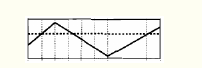cv2.THRESH_BINARY：大于阈值的部分被置为255，小于部分被置为0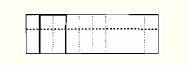cv2.THRESH_BINARY_INV：大于阈值部分被置为0，小于部分被置为255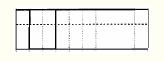cv2.THRESH_TRUNC：大于阈值部分被置为threshold，小于部分保持原样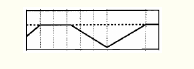cv2.THRESH_TOZERO：小于阈值部分被置为0，大于部分保持不变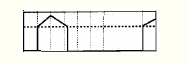cv2.THRESH_TOZERO_INV：大于阈值部分被置为0，小于部分保持不变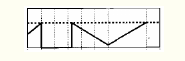其实还有很重要的 cv2.THRESH_OTSU：作为图像自适应二值化的一个很优的算法 Otsu 大津算法的参数：
使用为：cv2.threshold(img, 0, 255, cv2.THRESH_OTSU )
3 代码
import cv2

img1 = cv2.imread('./Image/cv.jpg', cv2.IMREAD_GRAYSCALE)

img1 = cv2.resize(img1, (300, 300), interpolation=cv2.INTER_AREA)
cv2.imshow('img1',img1)

ret,binary = cv2.threshold(img1,175,255,cv2.THRESH_BINARY)
ret,binaryinv = cv2.threshold(img1,175,255,cv2.THRESH_BINARY_INV)
ret,trunc = cv2.threshold(img1,175,255,cv2.THRESH_TRUNC)
ret,tozero = cv2.threshold(img1,175,255,cv2.THRESH_TOZERO)
ret,tozeroinv = cv2.threshold(img1,175,255,cv2.THRESH_TOZERO_INV)

cv2.imshow('binary',binary)
cv2.imshow('binaryinv',binaryinv)
cv2.imshow('trunc',trunc)
cv2.imshow('tozero',tozero)
cv2.imshow('tozeroinv',tozeroinv)
cv2.waitKey(0)

原图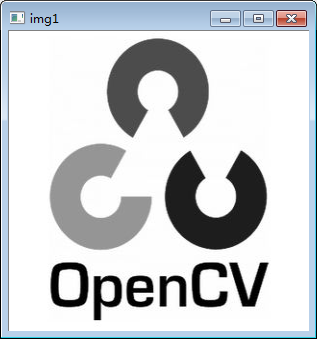1、cv2.THRESH_BINARY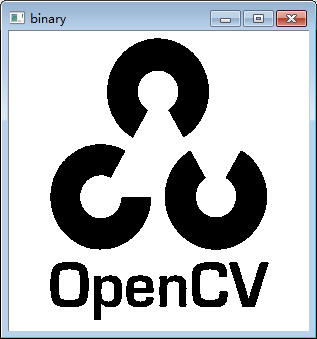2、cv2.THRESH_BINARY_INV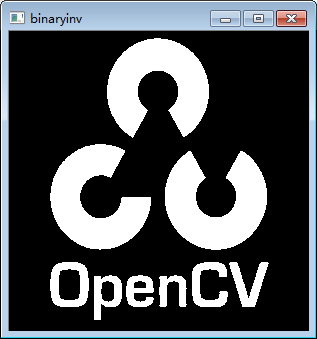3、cv2.THRESH_TRUNC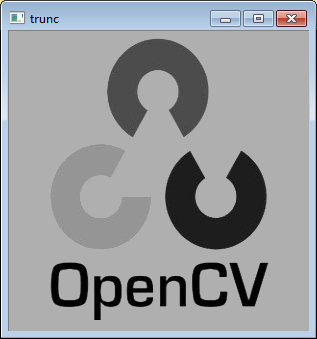4、cv2.THRESH_TOZERO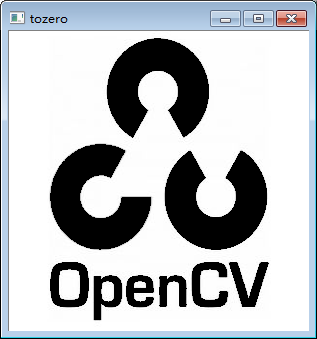5、cv2.THRESH_TOZERO_INV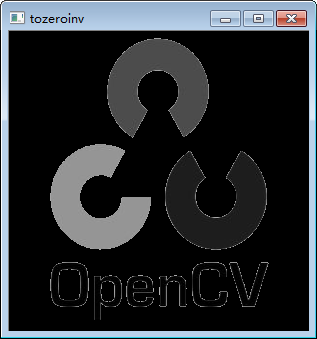十三 图片裁剪
1 opencv Rect()函数介绍
Rect(int _x,int _y,int _width,int _height);，参数意思为：左上角x坐标，左上角y坐标，矩形的宽，矩形的高。
一般的用法为：
Rect g_rectangle；
g_rectangle=Rect(a,b,c,d);

2 截取
本节内容来自这里。
本节内容来自这里。
十四 sobel边缘检测
import cv2
import numpy as np
import matplotlib.pyplot as plt

img = cv2.imread('number.jpg',0)
# 其中，0表示将图片以灰度读出来。

#### 图像边缘处理sobel细节
sobelx = cv2.Sobel(img, cv2.CV_64F, 1, 0, ksize=3)
# 利用Sobel方法可以进行sobel边缘检测
# img表示源图像，即进行边缘检测的图像
# cv2.CV_64F表示64位浮点数即64float。
# 这里不使用numpy.float64，因为可能会发生溢出现象。用cv的数据则会自动
# 第三和第四个参数分别是对X和Y方向的导数（即dx,dy），对于图像来说就是差分，这里1表示对X求偏导（差分），0表示不对Y求导（差分）。其中，X还可以求2次导。
# 注意：对X求导就是检测X方向上是否有边缘。
# 第五个参数ksize是指核的大小。

# 这里说明一下，这个参数的前四个参数都没有给谁赋值，而ksize则是被赋值的对象
# 实际上，这时可省略的参数，而前四个是不可省的参数。注意其中的不同点
# 还有其他参数，有需要的话可以去看，也可留言。

sobely = cv2.Sobel(img, cv2.CV_64F, 0, 1, ksize=3)
# 与上面不同的是对y方向进行边缘检测

sobelXY = cv2.Sobel(img, cv2.CV_64F, 1, 1, ksize=3)
# 这里对两个方向同时进行检测，则会过滤掉仅仅只是x或者y方向上的边缘

##### 图像展示
# 展示上面处理的图片，包括源图像。
# 注意使用subplot和title方法
plt.subplot(2,2,1)
plt.imshow(img,'gray')
# 其中gray表示将图片用灰度的方式显示，注意需要使用引号表示这是string类型。
# 可以用本行命令显示'gray'的类型：print(type('gray'))
plt.title('src')
plt.subplot(2,2,2)
plt.imshow(sobelx,'gray')
plt.title('sobelX')
plt.subplot(2,2,3)
plt.imshow(sobely,'gray')
plt.title('sobelY')
plt.subplot(2,2,4)
plt.imshow(sobelXY,'gray')
plt.title('sobelXY')
plt.show()https://blog.csdn.net/sunny2038/article/details/9170013
十五 插值技术
插值技术（Interpolate Technology）。是通过数学计算的方式。将两个值之间的部分进行平滑过渡的一种技术方案。
首先我们要明白什么叫做光滑的曲线，可以这么认为，这个曲线是一个运动物体，在时间[0，1]内运动的轨迹。而要求的光滑的曲线，就是要求物体运动过程中没有速度的突变。且要求不同的曲线段之间，速度也不能有突变。据此，我们可以大约知道插值一段曲线，需要知道曲线其实点的位置和速度，结束点的位置和速度。由于有四个已知变量，显然，用一个三次方程来描述这个曲线是再合适不过了。
十六 直线检测
https://blog.csdn.net/wishchin/article/details/83447861
https://blog.csdn.net/qq_19281769/article/details/84820515
https://blog.csdn.net/qq_19281769/category_8497640.html
https://www.cnblogs.com/WhyEngine/p/4020390.html
https://www.cnblogs.com/WhyEngine/p/4020294.html
https://blog.csdn.net/adamshan/article/details/78733302
https://blog.csdn.net/adamshan/category_7227853.html
https://adamshan.blog.csdn.net/article/details/78712120
https://blog.csdn.net/weixin_38746685/article/details/81613065
https://adamshan.blog.csdn.net/article/details/78712120
https://adamshan.blog.csdn.net/article/details/90578382
https://blog.csdn.net/qq_45281711/article/details/112980794
十七 角点检测
https://www.cnblogs.com/skyfsm/p/6899627.html
https://blog.csdn.net/zhu_hongji/article/details/81235643
https://www.cnblogs.com/ssyfj/p/9275368.html
https://blog.csdn.net/zhangquan2015/article/details/76686848
https://blog.csdn.net/piaoxuezhong/article/details/58587907
十八 曲线检测
https://blog.csdn.net/qq826309057/article/details/71328831
https://blog.csdn.net/zhinengshiyanshi/article/details/50500429
https://blog.csdn.net/u010996895/article/details/102939519


展开全文• 传统的图像质量客观评价方法主要包括均方误差（MSE，mean squared error）和峰值信噪比（PSNR，peak signal to noise rate）。均方误差法首先计算原始图像和失真像象素差值的均方值，然后通过均方值的大小来确定失真...
展开全文评价指标
• 本书分三部分（遥感数字图像处理基础、遥感数字图像质量改善、遥感数字图像特征选择与信息提取）由浅入深系统地介绍了遥感数字图像处理的原理与方法，其中不仅包括常用的经典方法，也包括近几年新提出的方法。...
• 在数字图像处理过程中，输入的是质量低的图像，输出的是改善质量后的图像， 常用的图像处理方法有图像增强、复原、编码、压缩等。 MATLAB既是一种直观、高效的计算机语言，同时又是一个科学计算平台。它为数据分析和...
• 常用的图像变换有傅里叶变换，DCT变换，小波变换。  图像增强：根据一定的要求，突出图像中感兴趣的信息，而减弱或去除不需要的信息，从而使有用信息得到加强的信息处理方法。图像增强主要方法有直方图
• 使用VC进行图像处理的时候，CBitmap类为我们提供了丰富位图处理函数，本文总结了该类相关函数和常用使用方法包括加载位图，显示位图，析构CBitmap资源以及在内存中保存位图等内容。
• 编程实现二值图像和灰度图像的几种常用的形态学处理方法包括：腐蚀、膨胀、开运算和闭运算。使用MFC完成界面编程，程序中包括：打开图像，显示图像，保存图像，参数输入和处理图像功能。
• 基本涵盖了常用的图像处理方法包括打开保存位jpg,tif,bmp等等，还有像指定大小缩放旋转，各种滤镜等等，亮度，色彩变换...是很好的参考程序！
• 这一小结，主要总结Opencv处理图像数据的方法包括图像的读取，变换和保存。 1.图像的读取 #导入opencv工具包，安装: pip install opencv-python import cv2 #导入图片 img = cv2.imread('D:\image_dataset\cat....opencv 计算机视觉
• 图像的阈值处理 一幅图像包括目标物体、背景还有噪声，要想从多值的数字图像中直接提取出目标物体，常用的方法就是设定一个阈值T，用T将图像的数据分成两部分：大于T的像素群和小于T的像素群。这是研究灰度变换的最...
• 介绍了变分图像分解图像处理方法的基本原理、常用的函数空间及变分图像分解模型。回顾了近几年变分图像分解图像处理方法在电子散斑干涉(ESPI)信息提取技术中的应用成果, 包括应用变分图像分解图像处理方法实现ESPI...
• 本程序包含数字图像处理的常用处理方法matlab实现，经过调试通过包括灰度处理，压缩编码，滤波等常见数字图像处理方法要求。
• 图像平滑方法包括空域法和频域法两大类。在空域法中，图像平滑常用的方法是采用均值滤波或中值滤波。   均值滤波 对于均值滤波，它是采用一个有奇数点的滑动窗口在图像上滑动，将窗口中心点对应的图像像素点的...opencv 计算机视觉
• 前言文本挖掘也是机器学习或者说是人工智能最需要处理的一类信息（其它诸如语音、图像及视频处理等）；随着数字信息化和网络化进程不断深入，用户在线交流、发布、共享等都被以文字形式记录下来，它们成为分析...
• 将介绍几种常用的图像变换的方法，即利用数学公式将图像变换成另一种具有特定物理意义的图像，通过新的图像，我们可以观察出原图像的某些特性，且可以对原图像进行滤波、压缩等图像处理的操作，包括傅里叶变换、...
• 摘自：http://blog.csdn.net/gamer_gerald/archive/2007/06/08/1643623.aspx NET framework框架类库中Image类和Bitmap类提供了常用图像格式解析读取和存储,这些常用格式包括BMP,JEPG,GIF,PNG,EXIF,TIFF....存储 null image path
• 标签图像文件格式（TagImageFileFormat，简写为TIFF）是一种灵活的位图格式，主要用来存储包括照片和艺术图在内的图像。扫描、传真、文字处理、光学字符识别和其它一些应用等都支持这种格式。今天小编就来和大家介绍...
• 基础知识汇总数字图像：数字图像处理包括内容：数字图像处理系统包括部分：从“模拟图像”到“数字图像”要经过步骤有：数字图像1600x1200什么意思？图像数字化包括哪两个过程？数字化图像数据量与哪些因素...
• 常用的方法包括低通滤波、高通滤波以及同态滤波等。 4.1 图像的对比度增强 4.1.1 灰度线性变换 1.灰度的线性变换 2.灰度分段线性变换 （1）扩展感兴趣的，牺牲其他 （2）扩展感兴趣的，压缩其他 4.1.2
• 主要包括数字图像处理技术介绍，相关算法，以及用VC实现的常用方法和示例。
• 基本涵盖了常用的图像处理方法包括打开保存位jpg,tif,bmp等等，还有像指定大小缩放旋转，各种滤镜等等，亮度，色彩变换...是很好的参考程序！
• 数字图像处理（Digital Image Processing） 是指用计算机处理图像，主要包括： （1）点运算：针对图像像素进行基本数学运算。点运算可以有效改变图像直方图分布，可以有效提高图像分辨率，以及图像均衡...算法 计算机视觉 vc++...